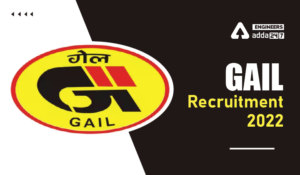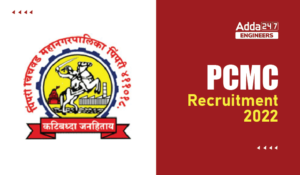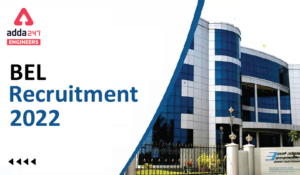Engineering Jobs   »   QUIZ FOR CIL MANAGEMENT TRAINEE FOR...

# QUIZ FOR CIL MANAGEMENT TRAINEE FOR MECHANICAL ENGINEER

Quiz contain 10 question with solution

time:15min

Q1. In spur Gear, circular pitch is equal to
(a) πD/N
(b) πN/D
(c) D/πN
(d) D/N

Q2. A rope drives a follower pully of radius 250 mm at 660 rad/min the coefficient of friction between the pulley & rope is 0.35. Find the velocity at which the rope drive in?
(a) 2.70 m/sec
(b) 2.60 m/sec
(c) 2.75 m/sec
(d) 3.10 m/sec

Q3. Measuring instrument is used to measure the atmospheric pressure
(a) piezometer
(b) pitote tube
(c) barometer
(d) thermometer

Q4. If basic size of a hole is 25 mm and fundamental deviation is 0.03 mm. the tolerance of the hole is 0.04 mm. find out the maximum diameter of hole.
(a) 25.03 mm
(b) 25.07 mm
(c) 25.04 mm
(d) 25.01 mm

Q5. Find out the work done during the process in which heat is supplied to the system is 100 KJ and change in internal energy is 20 KJ.
(a) 100 KJ
(b) 80 KJ
(c) 120 KJ
(d) 20 KJ

Q6. Find out the maximum moment for a cantilever beam having a point load of 5 KN at its free end and length of beam is 2m.
(a) 2 KNm
(b) 5 KNm
(c) 10 KNm
(d) 20 KNm

Q7. If a man is inside the lift going downward with acceleration of 4.9 m/sec². the mass of the man is 80 kg. find out the apparent weight of man.
(a) 392 N
(b) 1176 N
(c) 784.8 N
(d) 800 N

Q8. If a Gear is running at 80 rpm and pinion with 240 rpm it is also known that pinion having the 40 teeth find out the no. of teeth on gear wheel.
(a) 40 teeth
(b) 80 teeth
(c) 240 teeth
(d) 120 teeth

Q9. If height of manometer in mercury level is 10mm of Hg. If the manometer is a piezometer type find out the guage pressure.
(a) 1.36 Kpa
(b) 1.26 Kpa
(c) 1.33 Kpa
(d) 1.36 pa

Q10. If the net work output for a turbine power plat is 1800 KJ/kg. find out the specific steam consumption is –
(a) 2 kg/kwh
(b) 1 kg/kwh
(c) 1800 kg/kwh
(d) 3600 kg/kwh

Solutions

S1.
Ans.(a)
Sol.
πD/N

S2.
Ans.(c)
Sol.
V = πDN/60
V = rω but ω is in rad/min
V = 0.25 × 660/60
= 2.75 m/sec

S3.
Ans.(c)
Sol.
i.e. barometer

S4.
Ans.(b)
Sol.

Maximum diameter is 25 + 0.03 + 0.04
= 25.07 mm

S5.
Ans.(b)
Sol.
dQ = du + dw
dw = dQ – du
dw = 100 – 20
= 80 KJ

S6.
Ans.(c)
Sol.

Maximum moment is at the fixed end
M = 5 ×2 = 10 KNm

S7.
Ans.(a)
Sol.
Going downward than effective acceleration is (g+ay)
Therefore the apperant weight is W = m (g+ay)
= 80 [9.8 – 4.9]
= 392 N

S8.
Ans.(d)
Sol.
ω_G/ω_P =T_G/T_P
80/240=40/T_G
⇒ TG = 120 teeth

S9.
Ans.(a)
Sol.
Pressure P = ρgh
= 13.6 × 1000 × 10 × 0.01
= 1.36 Kpa

S10.
Ans.(a)
Sol.
Specific steam consumption is
= 3600/Wnet
= 3600/1800 kg/kwh
= 2 kg/kwh

Sharing is caring!

Thank You, Your details have been submitted we will get back to you.
•HPSC Lecturer Result 2022,
•How to Prepare for SSC JE 2022 General A...
•GAIL Recruitment 2022, GAIL 282 Non Exec...
•MP Vyapam Sub Engineer Notification 2022...
•PCMC Recruitment 2022, Check here the de...
•BEL Recruitment 2022, Check here For 13 ...